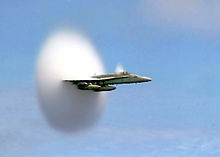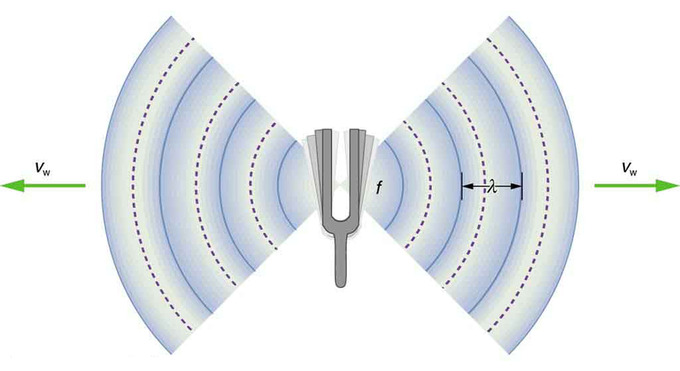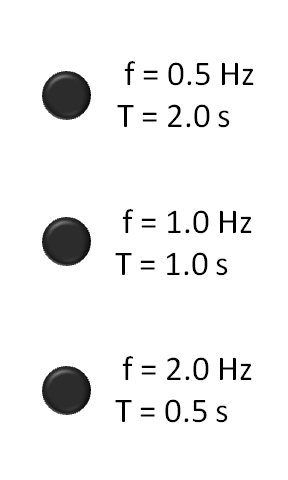$$\require{cancel}$$

# 16.1: Introduction

learning objectives

• Explain how humans can characterize sound

Before delving too far into the physics of calculating sound waves (studying things like the Doppler Effect, for example), lets address some basics of sound. Sound is a wave—a longitudinal wave of pressure that travels through compressible medias (i.e., solid, liquid, gaseous, or made of plasma ). There is no sound in a vacuum; by definition, a vacuum is a space free of any particles or matter. Thus there in a vacuum, there is no media through which sound waves can travel. Following are some characteristics of sound:

• Sound travels in longitudinal waves. When drawn these are also called sinusoidal waves, a visual example of which is shown in (we will cover this in more detail in a different section).
• Sound waves have frequency; that is, the pitch of sounds goes up or down.
• The amplitude of a sound determines its volume (loudness).
• Tone is a measure of the quality of a sound wave.
• Sound travels faster in a hot meduim, or in a solid. It also travels faster at sea level (where air pressure is higher).
• Sound intensity is the energy transmitted over a certain area. Intensity is a measure of the sound’s frequency.
• Ultrasound uses sound waves with high frequencies to see things normally hard to detect, like tumors. Animals, like bats and dolphins, use ultrasound (echolocation) to navigate and locate things. Ships also use a similar technique (known as SONAR) to locate things underwater. (This point will be discussed further in a more advanced Atom. )

## Sound Perception

Every sound wave has properties that define its frequency, such as wavelength, amplitude and intensity. Calculating these properties is outside the scope of this atom and will be addressed later. For now, it is important to know the basics of sound. As with light waves, sound frequencies have a range. Each living creature has a different level of sound perception. For example, consider the following examples of sound ranges (in Hz, Hertz):

• Humans 20 – 20,000 Hz
• Dogs 50 – 45,000 Hz
• Bats 20 – 120,000 Hz

By this comparison, humans have a relatively low sound perception.

## Sound SpeedBreaking the Sound Barrier: This familiar image is of a plane that is moving faster than the speed of sound.

As mentioned previously, the speed at which sound travels depends on the media through which the sound is traveling. It is much faster in a solid than in a liquid or gas. The general formula for calculating the speed of sound is given as:

$\mathrm{c=\sqrt{\dfrac{K}{ρ}},}$

where K is the coefficient of stiffness of the material (also called the Bulk modulus) and p is the density of the material. We will examine this further in another section. Generally, the expression ‘faster than the speed of sound’ refers to 344 m/s. is an image demonstrating a plane moving faster than the speed of sound. This general measurement is taken at sea level—at a temperature of 21 degrees Celsius under normal atmospheric conditions.

# Frequency of Sound Waves

Frequency is the number of occurrences of a repeating event per unit of time. The perception of frequency is called pitch.

learning objectives

• Relate frequency with the wavelength and speed of sound

Sound waves, like all other waves, have a property called frequency. Frequency is the number of occurrences of a repeating event per unit of time. The perception of frequency is called pitch.

Frequency is dependent on wavelength and the speed of sound. It is calculated with the following equation: $$\mathrm{f=\frac{v_s}{λ}}$$

This figure,, shows how the frequency is connected to wavelength.Frequency: A sound wave emanates from a source vibrating at a frequency f, propagates at v, and has a wavelength λ.

Alternatively, you can use the frequency and the wavelength to find the speed of sound in a specific medium. Remember that sound travels at different speeds in different mediums; sound moves fastest through a solid. The following equation is used to find the specific speed of sound, and is often easier to use than the standard speed of sound equation: vs=f∗λv_s=f*\lambda

A period is the duration of one cycle of a repeating event, and is the reciprocal or inverse of the frequency. The following animation shows different frequencies and their periods, from lowest to highest.Frequency Animation: Three flashing lights, from lowest frequency (top) to highest frequency (bottom). f is the frequency in hertz (Hz); or the number of cycles per second. T is the period in seconds (s); or the number of seconds per cycle. T and f are reciprocals.

## Hertz

The SI unit of frequency is called a Hertz, denoted Hz. A hertz is defined as the number of cycles per second. For example, 100 Hz signifies 100 cycles per second.

Different species can hear different frequency ranges. Humans can only hear from 20 Hz to 20,000 Hz, while dogs can hear up to 60,000 Hz. Bats can hear the highest ranges, up to 120,000 Hz. Bats use this super hearing, or ultrasound, to locate objects and prey. By bouncing sound waves off of another object and hearing how long it takes for the sound to echo back to them, they are able to approximate the distance between themselves and the object. This is called echolocation.

# Sound Production: Vibrating String and Air Columns

Sound can be produced by many different devices. A vibrating string or air column can both create music and have unique properties.

learning objectives

• Calculate the frequency of the sound wave produced by the string and a column of air

Sound can be produced in many ways, by many different instruments and devices. A vibrating string or air column can both make interesting music and it is good to understand the physics behind both.

## Vibrating Strings

There are many instruments that produce sound based on strings. Guitars, cellos, pianos and many other examples. These sounds are produced by standing waves in the strings. These waves and their frequencies are constant, and therefor the sound and pitch produced by them is constant. This figure shows a visual of a standing wave in a string: The speed of the wave is proportional to the root of the string tension, and inversely proportional to the root of the string density, shown by the following equation$$\mathrm{v=\sqrt{\frac{T}{μ}}}$$Pitch, and the way the sound is perceived depends on the frequency of the sound wave. Using the wave velocity, you can find the frequency using this equation: $$\mathrm{f=\frac{v}{2L}}$$, where L is the length of the string.Standing Waves in a String: Vibration, standing waves in a string. The fundamental and the first 6 overtones form a harmonic series.

## Air Columns

Many instruments make music by causing a vibration to a column of air in a resonator. These are usually called wind instruments. Examples of these are flutes, recorders, and saxophones. They are either open at both ends, or closed at one end and open at the other. The pitch of the sound is a function of the frequency. There are many factors that go into finding the frequency. Lets start with the tubes that are closed at one end and open at the other.

Closed Air Tubes:The maximum displacement of the air occurs at the open end of the tube, and is called the antinode. The air movement is constrained at the closed end, and there is no displacement, and this is called the node. The distance from the node to the antinode is 1/4 the length of one wavelength, and equal to the length of the tube, as shown in this equation:$$\mathrm{λ=4L}$$This can also be seen in this figure: The frequency is equal to the speed of sound in the air divided by the wavelength, or:$$\mathrm{f=\frac{v_w}{λ}=\frac{v_w}{4L}}$$, where vw is the speed of sound in the air, which we learned how to find in a previous atom.An Air column in a tube closed at one end: The fundamental and three lowest overtones for a tube closed at one end. All have maximum air displacements at the open end and none at the closed end.

Open Air tubes:Air tubes can also be open at both ends. They are very similar to the ones we talked about above, but there is an antinode at both ends, since they are both open, as shown in this figure: Since there is an antinode at both ends, we can see that the length of a wavelength is found by this equation: $$\mathrm{λ=2L}$$ And the frequency can be found in the following equation:$$\mathrm{f=\frac{v_w}{λ}=\frac{v_w}{2L}}$$

# Quality of Sound

Sound quality is an assessment of accuracy or enjoyability of how a sound is perceived.

Skills to Develop

• Identify factors that influence the sound quality of a reproduction of music

Sound quality is an assessment of accuracy or enjoyability of how a sound is perceived. It can be measured objectively or subjectively. What is the difference? Objective measurement is taken when tools are used to gauge accuracy. Subjective measurement is more of an opinion. When human listeners hear a sound and compare it to another sound they have heard, and decide which one they enjoy more, this is a subjective measurement of sound quality.

## Music Sound Quality

The quality of sound of live music is referred to as its tone. What makes this tone? Why does the same note being played on a piano sound different from that note played on a flute, or a guitar? When you hear a note, you mostly hear the fundamental frequency. But there are other harmonics present. You hear these, but they are much, much fainter than the fundamental, or main frequency. These are called overtones.

Each instrument has a different mix of these overtones, and this is why the sounds of similar notes sound different. The quality of this sound depends on these overtones to not take over what you are hearing. The overtones and fundamental frequency combine to make a unique frequency that you hear, which is illustrated in.Sound Frequencies: The quality of a tone depends on its mixture of harmonics.

## Reproduced Sound Quality

The sound quality of a reproduction of music depends on many factors. These include:

• Recording equipment
• Processing and mastering
• Reproduction equipment
• Listening environment

When you listen to a recording of music, you are hearing not only the music itself, but also any interference that may have been mistakenly recorded as well. The simplest form of digitally-stored audio is PCM.

As samples are placed closer together in time, higher frequencies can be reproduced. According to the sampling theorem, any signal with bandwidth B can be perfectly described by more than 2B samples per second. Audio must be sampled at above 40kHz: 44.1kHz for CD recordings and 48kHz for DVD recordings.

The amount of space required to store PCM depends on the number of bits per sample, the number of samples per second, and the number of channels. For CD audio, this is 44,100 samples per second, 16 bits per sample, and 2 channels for stereo audio leading to 1,411,200 bits per second.

# Speed of Sound

The speed of sound is is the distance traveled in a unit of time by a sound wave through an elastic medium, and is usually given as 344 m/s.

Skills to Develop

• Calculate the speed of sound from the properties of the media

## Speed of Soud

The speed of sound is is the distance traveled in a unit of time by a sound wave through an elastic medium. This medium can be a solid, liquid, gas or even plasma. The speed of sound is dependent on the properties of the media the sound is travelling through. The general value given for the speed of sound is the speed of a sound wave in air, at sea level, at normal atmospheric pressure; that number is 344 m/s. However, this number is not constant. Sound travels faster in a solid than in a liquid, and faster in a liquid that in a gas.

## Types of Sound Waves: Compression and Shear

There are two different kinds of sound waves: compression waves and shear waves. Compression waves can travel through any media, but shear waves can only travel through solids. The speed of a compression wave is determined by the media’s compression capacity, shear modulus, and density, while the speed of the shear wave is only determined by the shear modulus and density. The shear modulus is a measurement of the elasticity or rigidity of a material. Calculating this is outside of the scope of this atom, but there are tables which tell you its value for materials.

## Calculating the Speed of Sound

The speed of sound is usually denoted by cc, and a general equation can be used to calculate it. This is called the Newton-Laplace equation:c=√Kρc=KρK is the coefficient of stiffness, and p is the density of the media. From this equation, it is easy to see that the speed of sound will increase with stiffness and decrease with density. This is a very general equation, there are more specific derivations, for example:

The speed of sound in air at sea level is given by the following equation: $$\mathrm{c_{air}=331\frac{m}{s} \times \sqrt{\frac{T}{273K}}}$$ T is the temperature in Kelvin.

## Mach Number

You may have heard the term Mach number in relation to speed of space craft or jets before. This is a ratio of an object’s speed in relation to the speed of sound. The Mach number is given by the following, dimensionless equation: $$\mathrm{M=\frac{v}{a}}$$ – Mach numberv – Velocity of object a – Speed of sound in medium. If something is travelling at the speed of sound, that would make the equation equal to 1, and can be denoted as Mach 1. shows a jet that is travelling at the speed of sound or faster. The vapor cone is made just before it reaches the speed of sound and is caused by a sudden drop in air pressure.Faster than the Speed of Sound: This is a jet that is just about to break the sound barrier.

# Key Points

• Sound travels in longitudinal sinusoidal waves.
• Humans can characterize sound by frequency, amplitude and tone.
• The speed at which sound travels depends on the media through which the sound is traveling. It can be calculated using: $$\mathrm{c=\sqrt{\frac{K}{ρ}}}$$, where K is the coefficient of stiffness of the material (also called the Bulk modulus) and p is the density of the material.
• Frequency is dependent on wavelength and the speed of sound. It is calculated with the following equation: $$\mathrm{f=\frac{v_s}{λ}}$$
• A period is the duration of one cycle of a repeating event, and is the reciprocal or inverse of the frequency.
• The SI unit of frequency is called a Hertz, denoted Hz. A hertz is defined as the number of cycles per second. For example, 100 Hz signifies 100 cycles per second.
• The frequency, and therefore the pitch of a string instrument depends on the velocity of the sound wave and the length of the string. The frequency is found by: $$\mathrm{f=\frac{\sqrt{\frac{T}{μ}}}{2L}.}$$
• Sound in a tube of air that is closed at one end is found by the following equation: $$\mathrm{f=\frac{v_w}{λ}=\frac{v_w}{4L}}$$.
• Sound in a tube of air that is open at one end is found by the following equation: $$\mathrm{f=\frac{v_w}{λ}=\frac{v_w}{2L}}$$.
• Objective measurement is taken when tools are used to gauge accuracy. Subjective measurement is more of an opinion. When human listeners hear a sound and compare it to another sound they have heard, and decide which one they enjoy more, this is a subjective measurement of sound quality.
• The quality of sound of live music is referred to as its tone. When you hear a note, you mostly hear the fundamental frequency. But there are other harmonics present. You hear these, but they are much, much fainter than the fundamental, or main frequency. These are called overtones.
• The sound quality of a reproduction of music depends on many factors. These include: recording equipment, processing and mastering,reproduction equipment, and even listening environment.
• Sound can travel through any compressible material. These media can be solid, liquid, gas, or even plasma.
• The speed of sound is dependent on the properties of the material it travels through. It will travel faster through a solid than a liquid, and faster through a liquid than a gas.
• The general number given for the speed of sound is calculated at sea level, in air, at normal atmospheric pressure. That value is 344 m/s.

# Key Terms

• frequency: The quotient of the number of times n a periodic phenomenon occurs over the time t in which it occurs: $$\mathrm{f = \frac{n}{t}}$$.
• Hertz: Measurement of sound frequency.
• media: General term for different types of materials.
• period: The duration of one cycle in a repeating event.
• node: Point on a wave where there is no displacement.
• antinode: A region of maximum amplitude situated between adjacent nodes of a vibrating body, such as a string
• Subjective measurement: Based on a comparison to a previous experience, opinion.
• Objective measurement: Taken by tools to gauge accuracy.
• elasticity: The property by virtue of which a material deformed under the load can regain its original dimensions when unloaded
• kelvin: in the International System of Units, the base unit of thermodynamic temperature; 1/273.16 of the thermodynamic temperature of the triple point of water; symbolized as K